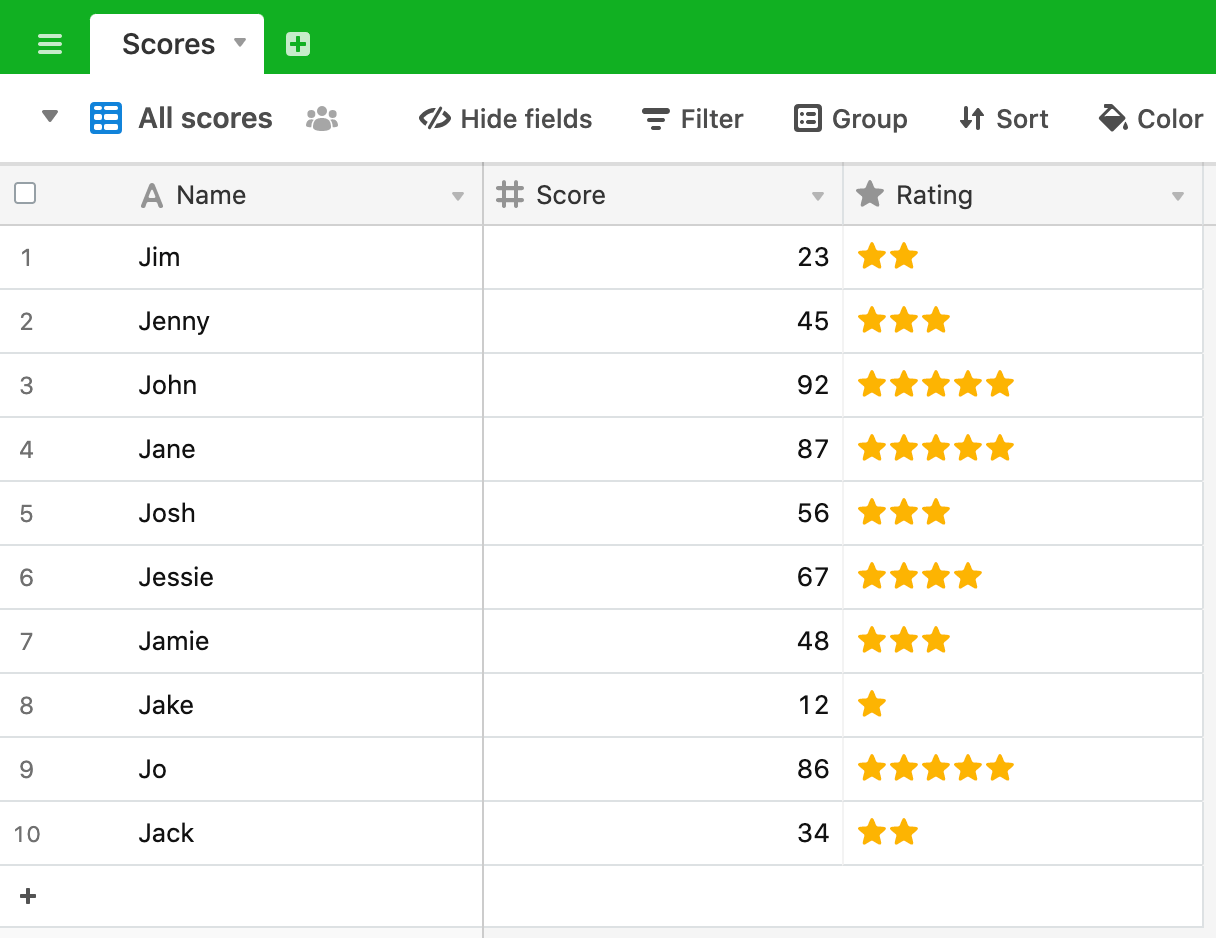# Converting scores to ratings

In this post, we’re going to look at how you can convert a score to a rating (using the Airtable “rating” field).

For this script, let’s say we’ve got a table something like this: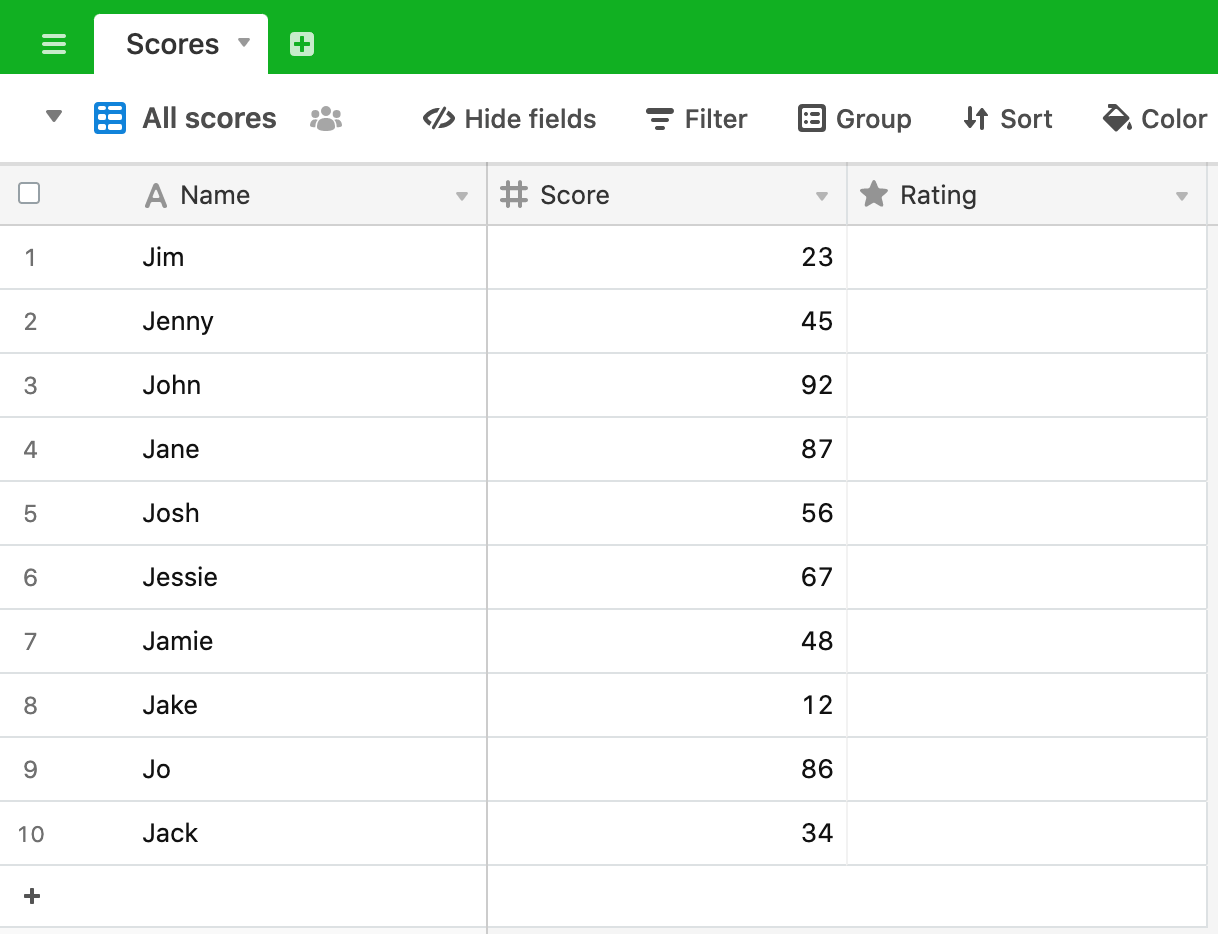Each person has a score (out of 100) and we want to convert this to a rating based on the following rating scheme:

1 - 20: ⭐️
21 - 40: ⭐️⭐️
41 - 60: ⭐️⭐️⭐️
61 - 80: ⭐️⭐️⭐️⭐️
81 - 100: ⭐️⭐️⭐️⭐️⭐️

When you define a rating field in Airtable you set the number of rating divisions (the default is 5), but, under the hood, the rating is simply stored as an integer.

We’ll build up our script step by step, testing scenarios as we go until we get the result we want. Intuitively, it seems like dividing the score by 5 is going to give us the answer, so let’s start there.

``````
let table = base.getTable('Scores');
let query = await table.selectRecordsAsync();

for (let record of query.records) {
let score = record.getCellValue('Score');
let rating = score/20;
console.log(score, rating)
}

``````

Here, we query the table and, for each record in the table, we get the `Score` and divide this by 5 to get the rating. We `console.log` the score and the rating to see the results.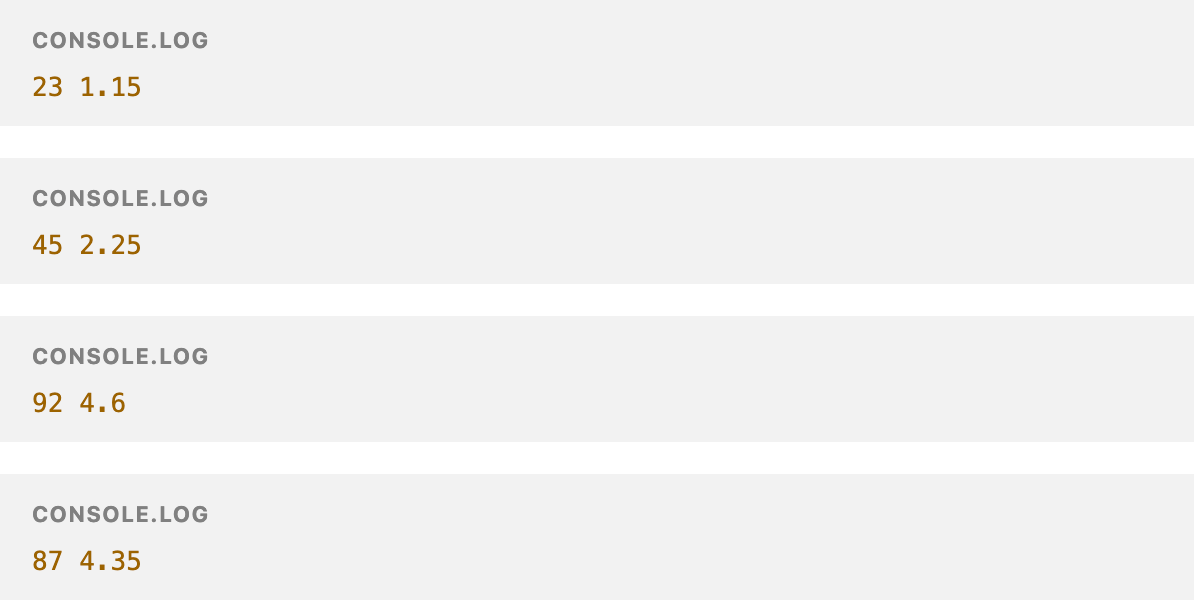We can immediately see that this isn’t right:

• The number is a decimal, not an integer
• The integer part of the rating is 1 less than we expected - 23 should score 2, but scores 1 and so on.

We can fix these two issues easily:

• We’ll use `Math.floor`, which returns the largest integer less than or equal to a given number, to remove the decimal element
• We’ll add 1 to the result to move the rating to the appropriate level.

Our script is now:

``````
let table = base.getTable('Scores');
let query = await table.selectRecordsAsync();

for (let record of query.records) {
let score = record.getCellValue('Score');
let rating = Math.floor(score/20) + 1;
console.log(score, rating)
}

``````

If we run this our results are a lot better: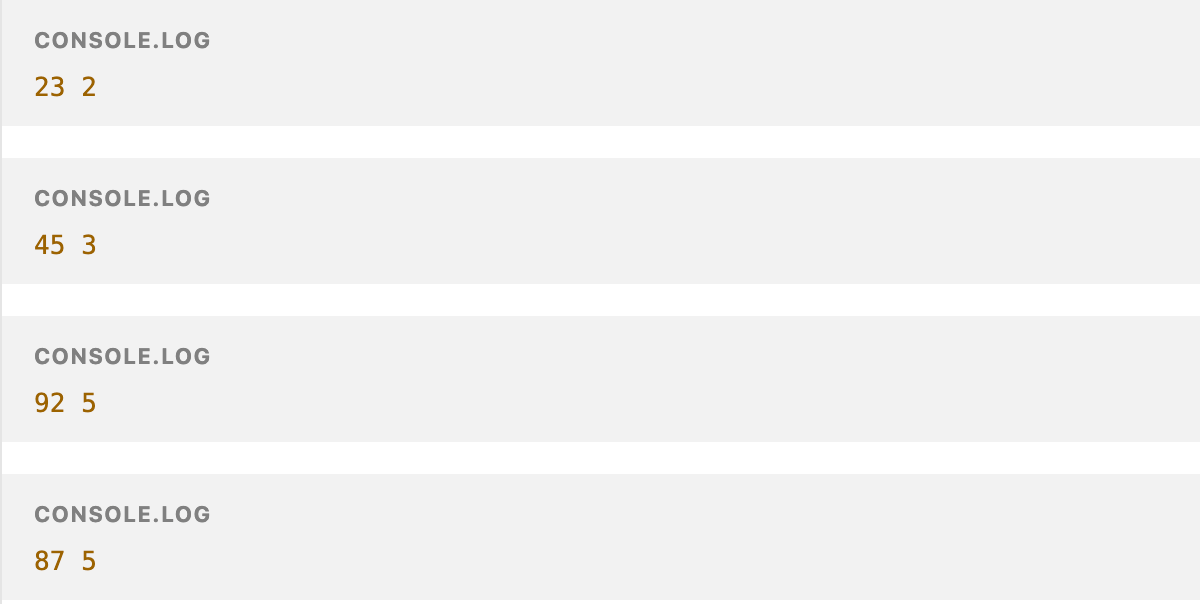On the face of it, this looks good, but we can check some other score values and their conversions to ratings to make sure. We can check the conversion for the minimum and maximum scores (0 and 100) and the conversion at a rating threshold (19, 20 and 21 for example).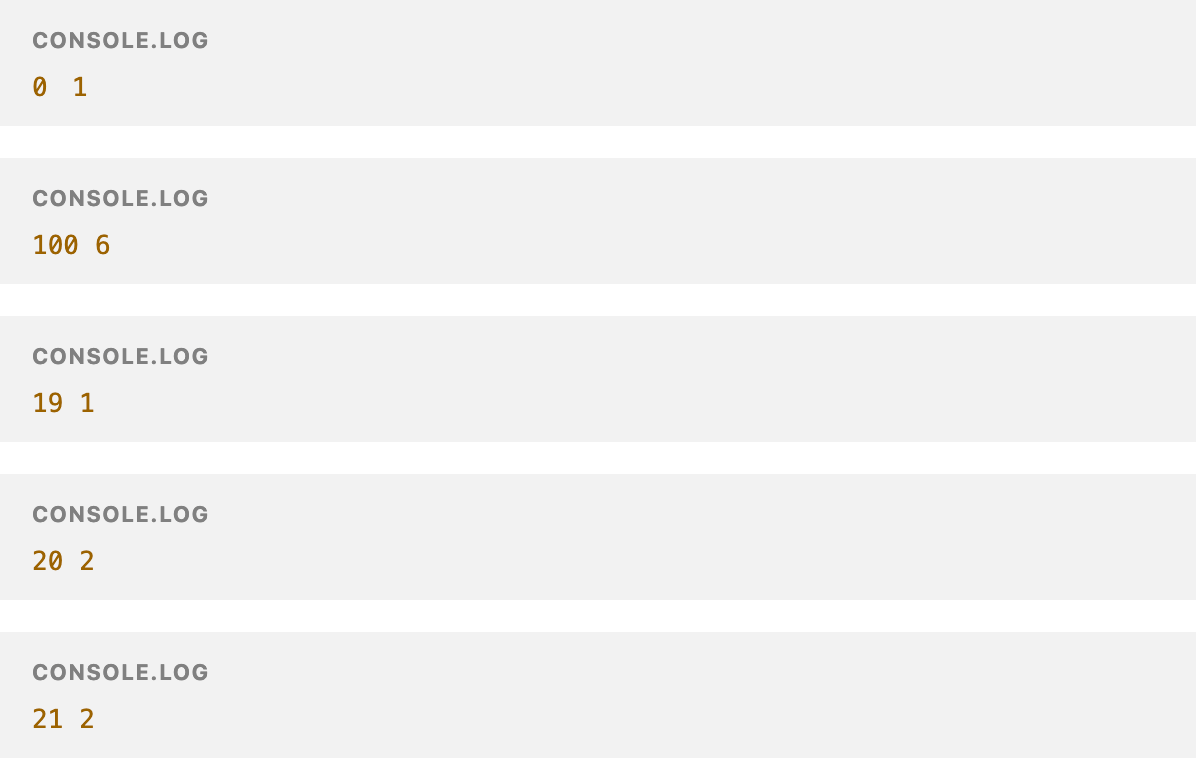Clearly, our script is not yet doing what we need it to do! A score of zero should result in a rating of zero (at least according to our scheme it shouldn’t get a rating of 1). A score of 100 should get a rating of 5. 19 and 21 have the correct rating, but we’re missing something at the top end of each boundary (20, 40, 60 etc).

The iteration of our script evaluates, not on `score`, but `score - 1`:

``````
let table = base.getTable('Scores');
let query = await table.selectRecordsAsync();

for (let record of query.records) {
let score = record.getCellValue('Score');
let rating = Math.floor((score-1)/20) + 1;
console.log(score, rating)
}

``````

When we run this we get:Better!

Now that our script is returning the values we want, we can set the Ratings value for each field using the `updateRecordAsync` method. Our final script is:

``````
let table = base.getTable('Scores');
let query = await table.selectRecordsAsync();

for (let record of query.records) {
let score = record.getCellValue('Score');
let rating = Math.floor((score-1)/20) + 1;
await table.updateRecordAsync(record, {
'Rating': rating
})
}

``````

And this gives us: